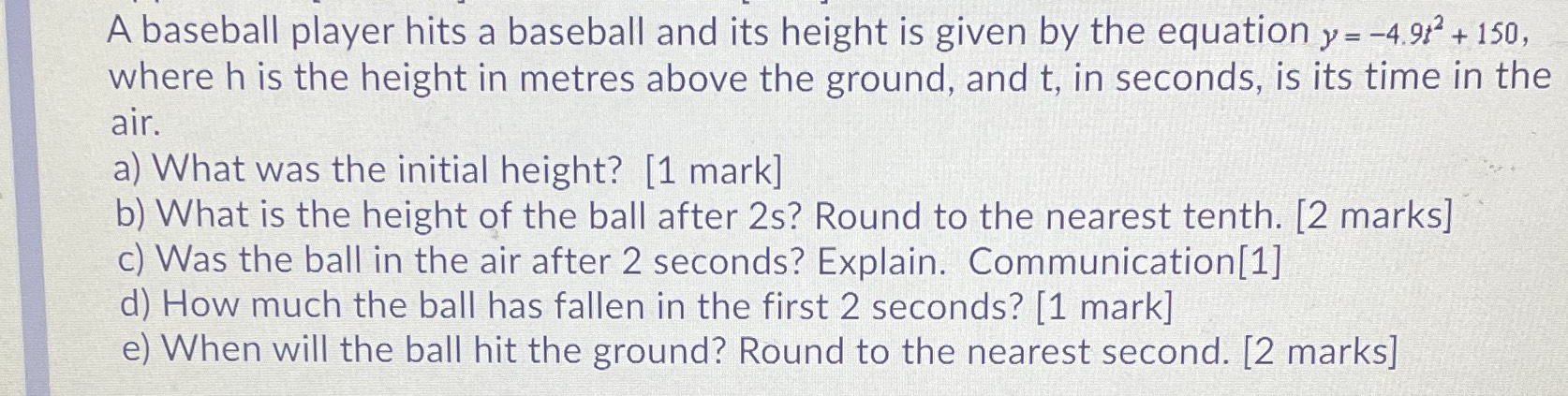### ¿Todavía tienes preguntas de matemáticas?

Pregunte a nuestros tutores expertos
Algebra
PreguntaA baseball player hits a baseball and its height is given by the equation $$y = - 4.9 t ^ { 2 } + 150 ,$$ where $$h$$ is the height in metres above the ground, and $$t$$ , in seconds, is its time in the  air.  a) What was the initial height? [1 mark]  b) What is the height of the ball after $$2 s$$ ? Round to the nearest tenth. [2 marks]  c) Was the ball in the air after $$2$$ seconds? Explain. Communication  d) How much the ball has fallen in the first $$2$$ seconds? [1 mark]  e) When will the ball hit the ground? Round to the nearest second. [2 marks]